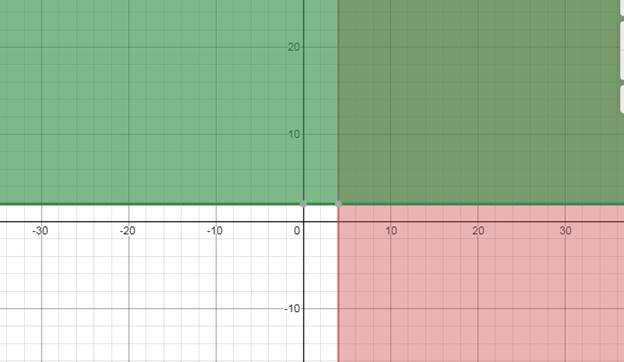# To graph : The region is given by : { ( x , y ) | x ≥ 4 o r y ≥ 2 }### Precalculus: Mathematics for Calcu...

6th Edition
Stewart + 5 others
Publisher: Cengage Learning
ISBN: 9780840068071### Precalculus: Mathematics for Calcu...

6th Edition
Stewart + 5 others
Publisher: Cengage Learning
ISBN: 9780840068071

#### Solutions

Chapter 1, Problem 102RE
To determine

## To graph: The region is given by : {(x,y)|x≥4ory≥2}

Expert Solution

### Explanation of Solution

Given information:

The region is given by : {(x,y)|x4ory2}

Graph:

In order to solve an equation graphically, find all the values that satisfy the equation. To solve an equation graphically, draw the graph for each side, member, of the equation and see where the curves cross, are equal. The x values of these points, are the solutions to the equation.

The overlapped region or the common region shows the region given by the above set.Interpretation:

In order to solve an equation graphically, find all the values that satisfy the equation. To solve an equation graphically, draw the graph for each side, member, of the equation and see where the curves cross, are equal. The x values of these points, are the solutions to the equation

The overlapped region or the common region shows the region given by the above set.

### Have a homework question?

Subscribe to bartleby learn! Ask subject matter experts 30 homework questions each month. Plus, you’ll have access to millions of step-by-step textbook answers!